Need help? Email help@shout.education

# Complex Ions – Acidity of the Hexaaqua Ions

This page explains why complex ions of the type [M(H2O)6]n+ are acidic.

## The General Explanation

### A Closer Look at the Distribution of Charge in the Ion

The pH's of solutions containing hexaaqua ions vary a lot from one metal to another (assuming you are comparing solutions of equal concentrations). However, the underlying explanation is the same for all of them.

We'll take the hexaaquairon(III) ion, [Fe(H2O)6]3+ as typical. The structure of the ion is: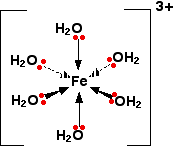Each of the six water molecules are attached to the central iron(III) ion via a co-ordinate bond using one of the lone pairs on the oxygen.

Note: This bonding is explained in some detail on the introductory page on complex ions. Follow this link if you aren't sure.

We'll choose one of these water molecules at random (it doesn't make any difference which one!), and look at the bonding in a bit more detail – showing all the bonds around the oxygen.

Imagine for the moment that the 3+ charge is located entirely on the iron.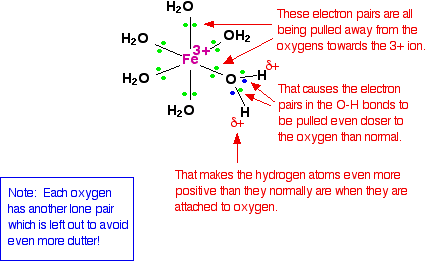When the lone pairs on the oxygens form co-ordinate bonds with the iron, there is obviously a movement of electrons towards the iron.

That has an effect on the electrons in the O-H bonds. These electrons, in turn, get pulled towards the oxygen even more than usual. That leaves the hydrogen nuclei more exposed than normal.

The overall effect is that each of the hydrogen atoms is more positive than it is in ordinary water molecules. The 3+ charge is no longer located entirely on the iron but spread out over the whole ion – much of it on the hydrogen atoms.

### The Effect of Dissolving This Ion in Water

#### The theory

The hydrogen atoms attached to the water ligands are sufficiently positive that they can be pulled off in a reaction involving water molecules in the solution.

The first stage of this process is:

\left[Fe(H_2O)_6\right]^{3+} + H_2O \xrightleftharpoons{} \left[Fe(H_2O)_5(OH)\right]^{2+} + H_3O^+

The complex ion is acting as an acid by donating a hydrogen ion to water molecules in the solution. The water is, of course, acting as a base by accepting the hydrogen ion.

Because of the confusing presence of water from two different sources (the ligands and the solution), it is easier to simplify this:

\left[Fe(H_2O)_6\right]^{3+}_{(aq)} \xrightleftharpoons{} \left[Fe(H_2O)_5(OH)\right]^{2+}_{(aq)} + H^+_{(aq)}

However, if you write it like this, remember that the hydrogen ion isn't just falling off the complex ion. It is being pulled off by a water molecule in the solution. Whenever you write "H+(aq)" what you really mean is a hydroxonium ion, H3O+.

The hexaaquairon(III) ion is quite strongly acidic giving solutions with pH's around 1.5, depending on concentration. You can get further loss of hydrogen ions as well, from a second and a third water molecule.

Losing a second hydrogen ion:

\left[Fe(H_2O)_5(OH)\right]^{2+}_{(aq)} \xrightleftharpoons{} \left[Fe(H_2O)_4(OH)_2\right]^{+}_{(aq)} + H^+_{(aq)}

and a third one:

\left[Fe(H_2O)_4(OH)_2\right]^{+}_{(aq)} \xrightleftharpoons{} \left[Fe(H_2O)_3(OH)_3\right]_{(s)} + H^+_{(aq)}

This time you end up with a complex with no charge on it. This is described as a neutral complex. Because it has no charge, it doesn't dissolve in water to any extent, and a precipitate is formed.

#### In practice

What do you actually get in solution if you dissolve an iron(III) salt in water? In fact you get a mixture of all the complexes that you have seen in the equations above. These reactions are all equilibria, so everything will be present. The proportions depend on how concentrated the solution is.

The colour of the solution is very variable and depends in part on the concentration of the solution. Dilute solutions containing iron(III) ions can be pale yellow. More concentrated ones are much more orange, and may even produce some orange precipitate.

None of these colours represents the true colour of the [Fe(H2O)6]3+ ion – which is a very pale lilac colour! That colour is only really easy to see in solids containing the ion.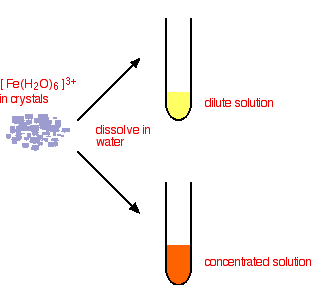Looking at the equilibrium showing the loss of the first hydrogen ion:

\underset{\text{pale lilac}}{\left[Fe(H_2O)_6\right]^{3+}} + \color{#467abf}{H_2O} \xrightleftharpoons{} \underset{\text{orange}}{\left[Fe(H_2O)_5(OH)\right]^{2+}} + \color{#467abf}{H_3O^+}

The colour of the new complex ion on the right-hand side is so strong that it completely masks the colour of the hexaaqua ion.

In concentrated solutions, the equilibrium position will be even further to the right-hand side (Le Chatelier's Principle), and so the colour darkens. You will also get significant loss of other hydrogen ions leading to some formation of the neutral complex – and so you get some precipitate.

Note: This is a bit of a simplification, but adequate for this level. In fact you get other changes happening to the new complex ions as well. For example, the first one dimerises – introducing further equilibria and colours! Don't worry about it!

You can move the position of this equilibrium by adding extra hydrogen ions from a concentrated acid – for example, by adding concentrated nitric acid to a solution of iron(III) nitrate.

The new hydrogen ions push the position of the equilibrium to the left so that you can see the colour of the hexaaqua ion. This is slightly easier to follow if you write the simplified version of the equilibrium.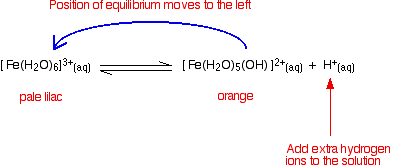Note: I haven't tried to draw this colour change because when I last tried this I couldn't make it work properly (although I have done in the past)! The solution concentration is quite critical. If it is too dilute, the yellow colour disappears and you are left with a solution which is so faintly lilac that it is (in all honesty!) colourless. If the solution is too concentrated, you never get rid of the strong orange colour of the other complex ions, and so the lilac colour remains masked.

## Why are 3+ Ions More Acidic Than 2+ Ions

### The Effect of Charge on the Acidity of the Hexaaqua Ions

Solutions containing 3+ hexaaqua ions tend to have pH's in the range from 1 to 3. Solutions containing 2+ ions have higher pH's – typically around 5 – 6, although they can go down to about 3.

Remember that the reason that these ions are acidic is because of the pull of the electrons towards the positive central ion. An ion with 3+ charges on it is going to pull the electrons more strongly than one with only 2+ charges.

In 3+ ions, the electrons in the O-H bonds will be pulled further away from the hydrogens than in 2+ ions.

That means that the hydrogen atoms in the ligand water molecules will have a greater positive charge in a 3+ ion, and so will be more attracted to water molecules in the solution.

If they are more attracted, they will be more readily lost – and so the 3+ ions are more acidic.

## The Effect of Ionic Radius on Acidity

### Charge Density and Acidity

If you have ions of the same charge, it seems reasonable that the smaller the volume this charge is packed into, the greater the distorting effect on the electrons in the O-H bonds.

Ions with the same charge but in a smaller volume (a higher charge density) would be expected to be more acidic.

You would therefore expect to find that the smaller the radius of the metal ion, the stronger the acid. Unfortunately, it's not that simple!

Warning! If you like your chemistry all tidy and simple, don't read any further. I have got to the point that most text books get to at this level. In fact, as I will show below, it is quite difficult to show this effect of ionic radius in any simple ions. There are too many exceptions.

### Plotting pKa Values Against Ionic Radius

#### pKa

pKa is a scale for comparing the strengths of weak acids. All you really need to know for the present topic is that the lower the value of pKa, the stronger the acid. As a reasonable approximation, if you divide the pKa value of a weak acid by 2, that will give you the pH of a 1 mol dm-3 solution of the acid.

Note: It isn't necessary for this topic, but if you need to know more about pKa, you can find it by following this link.

A real problem arises here! Almost every data source that you refer to quotes different values for ionic radii. This is because the radius of a metal ion varies depending on what negative ion it is associated with. We are interested in what happens when the metal ion is bonded to water molecules, so haven't got simple ions at all! Whatever values we take are unlikely to represent the real situation.

In the graphs which follow I have taken values for ionic radii from two common sources so that you can see what a lot of difference it makes to the argument.

#### The graphs

If it is true that the smaller the ionic radius, the stronger the acidity of the hexaaqua ion, you would expect some sort of regular increase in pKa (showing weaker acids) as ionic radius increases.

The following graphs plot pKa against ionic radii for the 2+ ions of the elements in the first transition series from vanadium to copper.

The first graph plots pKa against ionic radii taken from Chemistry Data Book by Stark and Wallace.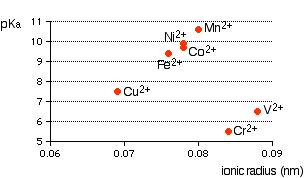You can see that there is a trend for several of the ions, but it is completely broken by vanadium and chromium.

The second graph uses ionic radii taken from the Nuffield Advanced Science Book of Data.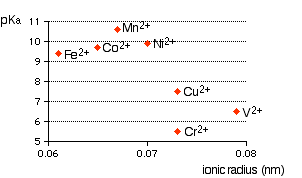In this case, the pattern is much more confused. Which is right? I don't know!!

### A Final Comment

During the couple of weeks I spent trying to understand this, I failed completely. The only conclusion I came to is that there probably is a relationship between ionic radius and acid strength, but that it is nothing like as simple and straightforward as most books at this level pretend.

The problem is that there are other more important effects operating as well (quite apart from differences in charge) and these can completely swamp the effect of the changes in ionic radius. You have to look in far more detail at the bonding in the hexaaqua ions and the product ions. This is all just too difficult to understand at this level. In fact, to be honest, I did wonder while I was researching this whether anyone really understood all of this! There is, of course, no reason why they should – it is nonsense to think that we can explain everything in chemistry at the present moment.